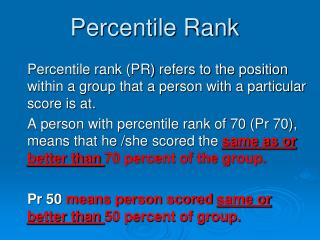DownloadDownload PresentationPercentile Rank

# Percentile Rank

Télécharger la présentation## Percentile Rank

- - - - - - - - - - - - - - - - - - - - - - - - - - - E N D - - - - - - - - - - - - - - - - - - - - - - - - - - -
##### Presentation Transcript

1. Percentile Rank Percentile rank (PR) refers to the position within a group that a person with a particular score is at. A person with percentile rank of 70 (Pr 70), means that he /she scored the same as or better than 70 percent of the group. Pr 50 means person scored same or better than 50 percent of group.

2. Percentile Rank – data on handout answers the question • What percentile rank tells us is within this group what position a baby’s weight represents. • 8 lbs For example ? • And gives us some idea if this is high or low relative to group

3. Percentile Rank - data on handoutbirth weights Step One – list the weights (or scores)

4. Percentile Rank - data on handoutbirth weights (30 babies) Step Two – how many births (or scores) at each weight

5. Percentile Rank – Relative Frequency Percent • Step Three – calculate what the number at each score represents of the whole • If 5 out of the 30 weigh 8 lbs, what does that weight represent as a percentage of the whole (30) ?

6. Percentile Rank - data on handoutbirth weights (30 babies) Step Three – relative frequency percent

7. Percentile Rank - data on handoutbirth weights (30 babies) Step Four – cumulative percent (Percentile Rank)

8. Percentile Rank • A baby weighing 8 lbs amongst this group (births in a week in Pleasantville) would be • Pr87 (at the 87th percentile) • Which is the same (weight) as, or better than 87 % of the group (babies born that week).